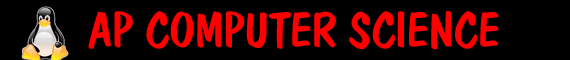Math projects:   In a class called MathProjects   1. Create a method called void thirdRoot (double x) that will print out the 3rd root of a number   2. Using random, write a method randomHeight that will print a a random height for a man between 5'2 and 6'4. (all equally likely)   3. Create a method called int yearsFromMyAge(int theirAge) that when given the user's age it will return how many years they are from your age. Use MATH.abs   4.Create a method that will public double findWeight(int currentHeight, int currentWeight, int otherHeight) that will find how much the person would weigh if they were in the same proportion but of a different height. The formula is otherWeight equals otherHeight divided by currentHeight all to the 3rd power, times currentWeight.   EC 5. Create a method public double findThirdSide(double a, double b, double C), given two sides and included angle, find and return the third side. Link   EC 6.: Create a method void dueDate(int month, int day, int year) that will calculate a baby's due date based on their date of conception. A child is born 40 weeks from conception.   ``` public class MathProjects { public void thirdRoot (double x) { } public void randomHeight () { } public int yearsFromMyAge(int theirAge) { return 0; } public double findWeight(int currentHeight, int currentWeight, int otherHeight) { return 0; } public static void main (String[] args) { MathProjects m = new MathProjects(); m.thirdRoot(27); m.randomHeight(); System.out.println("You are " + m.yearsFromMyAge(37) + " years different then me"); System.out.println("You would weigh " + m.findWeight(75,175, 65)+ " pounds."); } } ```